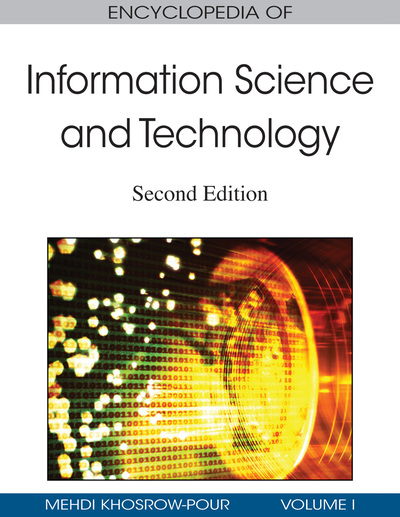# Robustness in Neural Networks

Cesare Alippi (Politecnico di Milano, Italy), Manuel Roveri (Politecnico di Milano, Italy) and Giovanni Vanini (Politecnico di Milano, Italy)
DOI: 10.4018/978-1-60566-026-4.ch527

## Abstract

The robustness analysis for neural networks aims at evaluating the influence on accuracy induced by perturbations affecting the computational flow; as such it allows the designer for estimating the resilience of the neural model w.r.t perturbations. In the literature, the robustness analysis of neural networks generally focuses on the effects of perturbations affecting biases and weights. The study of the network’s parameters is relevant both from the theoretical and the application point of view, since free parameters characterize the “knowledge space” of the neural model and, hence, its intrinsic functionality. A robustness analysis must also be taken into account when implementing a neural network (or the intelligent computational system into which a neural network is inserted) in a physical device or in intelligent wireless sensor networks. In these contexts, perturbations affecting the weights of a neural network abstract uncertainties such as finite precision representations, fluctuations of the parameters representing the weights in analog solutions (e.g., associated with the production process of a physical component), ageing effects or more complex, and subtle uncertainties in mixed implementations.
Chapter Preview
Top

## Background

The sensitivity/robustness issue has been widely addressed in the neural network community with a particular focus on specific neural topologies. In particular, when the neural network is composed of linear units, the relationship between perturbations and the induced performance loss can be obtained in a closed form (Alippi & Briozzo, 1998). Conversely, when the neural topology is non-linear, we have either to assume the small perturbation hypothesis or particular assumptions about the stochastic nature of the neural computation (e.g., see Alippi (2002a), Alippi et al. (1998), and Pichè, 1995); unfortunately, such hypotheses are not always satisfied in real applications. Another classic approach requires expanding the neural computation with Taylor around the nominal value of the trained weights. A subsequent linearized analysis follows, which allows the researcher to solve the sensitivity issue problem (Pichè, 1995). This last approach has been widely used in the implementation design of neural networks where the small perturbation hypothesis abstracts small errors introduced by finite precision representations of the weights (Dundar & Rose, 1995; Holt & Hwang, 1993). Again, the validity of the analysis depends on the validity of the small perturbation hypothesis.

Differently, other authors avoid the small perturbation assumption by focusing the attention on very specific neural network topologies and/or by introducing particular assumptions regarding the distribution of perturbations, internal neural variables, and inputs as done for Madalines neural networks (Alippi, Piuri, & Sami, 1995; Stevenson, Winter, Widrow, 1990).

Some other authors tackle the robustness issue differently by suggesting techniques leading to neural networks with improved robustness ability by acting on the learning phase (e.g., see Alippi, 1999) or by introducing modular redundancy (Edwards & Murray, 1998); though, no robustness indexes are suggested there. The robustness of neural networks with respect to hardware implementations were also studied in Hereford and Kuyucu (2005) and Nugent, Kenyon, and Porter (2004) where authors proposed evolutionary and adaptive approaches.

Again, the study of robustness over training time has been evaluated for neural networks in the large, without assuming the small perturbation hypothesis (Alippi, Sana, & Scotti, 2004). In this direction, other authors have addressed the issue of the robustness analysis during the training phase (Manic & Wilamowski, 2002; Qin Juanyin, Wei Wei, & Wang Pan, 2004) by suggesting a genetic approach or by considering the use of the regression theory.

## Key Terms in this Chapter

Wireless Sensor Network: A network of distributed sensors linked by wireless connections.

Randomized Algorithms: A probabilistic sampling technique for exploring a space combining learning theories and Monte Carlo approaches.

Neural Network Weights: The free parameters of the neural model.

Knowledge Space: The space defined by the neural network weights.

Robustness: A property possessed by a system. A system is robust with respect to a perturbation when the perturbation effect on the system performance is tolerable according to a predefined threshold.

## Complete Chapter List

Search this Book:
Reset